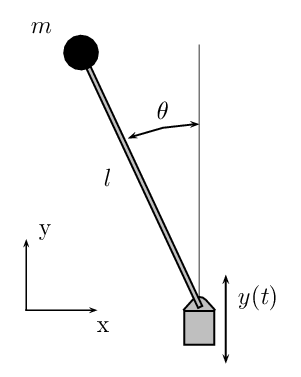# Inverted pendulumThe inverted pendulum has Lagrangian$Latex formula$, and equation$Latex formula$ where$Latex formula$ is the angle to the vertical. Alternatively, let$Latex formula$ be the Cartesian coordinates of the position of the pendulum, subject to the constraint$Latex formula$.
The Lagrangian is then$Latex formula$

and the mechanical equations are$Latex formula$

where we have set an horizontal force as the control$Latex formula$.
We want to minimize the objective$Latex formula$

Figure 1 shows the states$Latex formula$, the control$Latex formula$ and multiplier$Latex formula$.

Numerical simulations:
Discretization: Runge Kutta 4 with 400 steps.
We take here$Latex formula$,$Latex formula$ and$Latex formula$.
The final conditions are$Latex formula$

The initial conditions are$Latex formula$